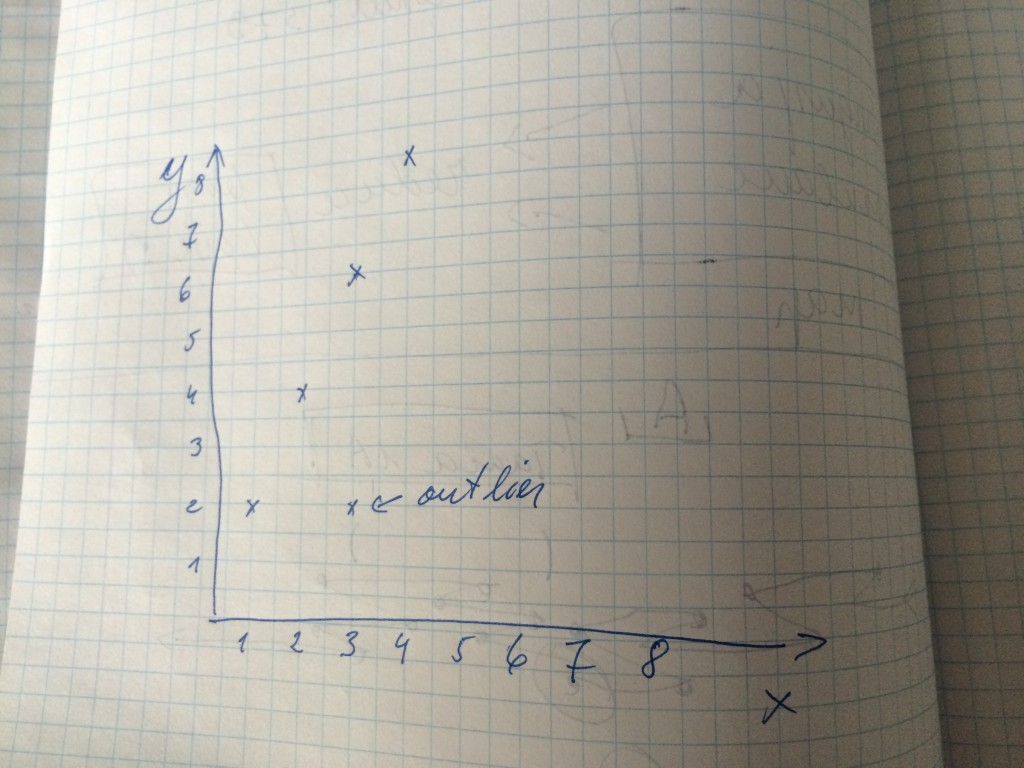# Multivariate data detect outliers with mahout and Mahalanobis distance algorithm

I am not mathematician but one of our project needed that we will find outliers from multivariate population. As I understand Mahalanobis distance is one widely used algorithm.  This is example with very simple dataset to show distance between normal points and outlier.

So my simple dataset is two dimension so we can display it in x;y graph:

{1;2, 2;4, 3;6, 3;2, 4;8}

Let’s put it into paperthe outlier is clearly visible – 3;2

Now I use mahout MahalanobisDistanceMeasure package (org.apache.mahout.common.distance.MahalanobisDistanceMeasure)

package com.deciderlab.MahalanobisDistanceMeasure;

import org.apache.mahout.common.distance.MahalanobisDistanceMeasure;

import org.apache.mahout.math.Matrix;

import org.apache.mahout.math.RandomAccessSparseVector;

import org.apache.mahout.math.SparseMatrix;

import org.apache.mahout.math.Vector;

publicclass DistanceMahalanobisSample {

publicstaticvoid main(String[] args) {

double[][] d = { { 1.0, 2.0 }, { 2.0, 4.0 },

{ 3.0, 6.0 }, { 3.0, 2.0 }, { 4.0, 8.0 } };

Vector v1 = new RandomAccessSparseVector(2);

v1.assign(d);

Vector v2 = new RandomAccessSparseVector(2);

v2.assign(d);

Vector v3 = new RandomAccessSparseVector(2);

v3.assign(d);

Vector v4 = new RandomAccessSparseVector(2);

v4.assign(d);

Vector v5 = new RandomAccessSparseVector(2);

v5.assign(d);

Matrix matrix = new SparseMatrix(2, 2);

matrix.assignRow(0, v1);

matrix.assignRow(1, v2);

double distance1;

double distance2;

MahalanobisDistanceMeasure dmM = new MahalanobisDistanceMeasure();

dmM.setInverseCovarianceMatrix(matrix);

distance0 = dmM.distance(v2, v1);

distance1 = dmM.distance(v2, v3);

distance2 = dmM.distance(v2, v4);

System.out.println(“d0=” + distance0 +  ” ,d1=” + distance1 + “, d2=” + distance2);

}

}

Compile it. I use maven to deal with dependencies.

Run it:

[margusja@vm37 MahalanobisDistanceMeasure]\$ hadoop jar /var/www/html/margusja/MahalanobisDistanceMeasure/target/MahalanobisDistanceMeasure-1.0-SNAPSHOT.jar com.deciderlab.MahalanobisDistanceMeasure.DistanceMahalanobisSample

d0=5.0 ,d1=5.0, d2=3.0

So, distance between v1 (1;2) and v2 (2;4) is 5.0 and distance between v2 (2;4) and v3(3;6) is 5.0 but distance between v2(2;4) and v4 (3;2) is 3.0. So it allows me mark record (3;2) mark as outlier.